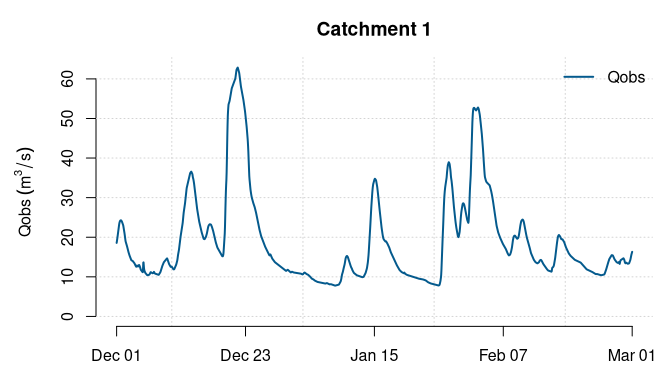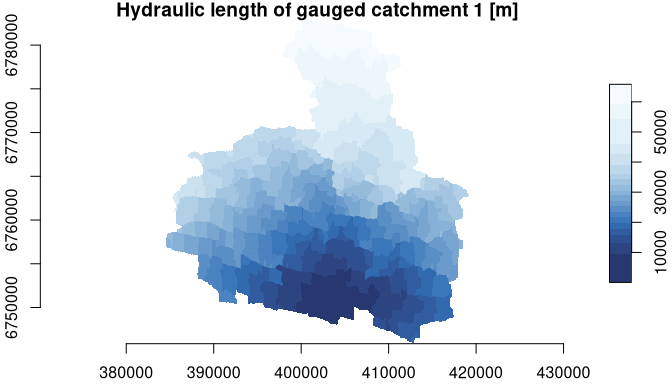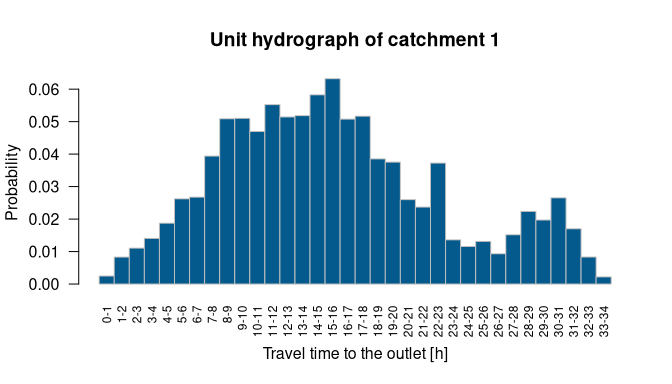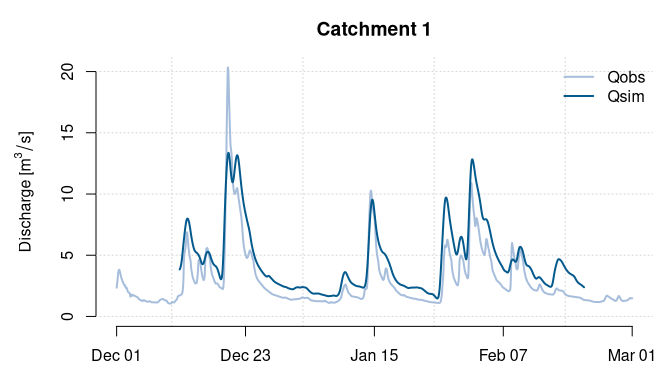# Get started with transfR

#### 2022-05-11

This package aims to estimate discharge time series of ungauged catchments (non-instrumented catchment where discharge is therefore not available) using hydrological observation of neighbouring gauged catchments (instrumented catchment where discharge is available). The hydrological modelling is based on a description of catchment geomorphology that can be assessed at any location. An inversion of this model at gauged locations allows estimating the net rainfall that makes the transfer of observed discharge easier to ungauged locations.

## 1. Create a transfR object

An object of class transfR needs to be created first with the function as_transfr(). It will also be used to gather all the catchment attributes and intermediary results from the different steps. This object needs to be created from two users inputs:

• a spatio-temporal object (stars object) that is describing both discharge and the corresponding spatial support (outlet, centroid or catchment boundary).
• raster maps of hydraulic length (stars or matrix object) for each catchment describing the flow path length from each pixel to the outlet within the river network (Cudennec et al. 2004; Aouissi et al. 2013).

This package does not provide functions to create these two inputs. It needs to be prepared beforehand by the user. Several GIS softwares offer possibilities to extract them from a digital elevation model such as GRASS toolkits (Jasiewicz and Metz 2011), Whitebox GAT (see Lindsay (2016) or WhiteboxTools), TauDEM (D. Tarboton, Utah State University) or online services (Squividant et al. (2015) for catchment delineation only).

The ‘Oudon’ example dataset contains these two inputs with hourly discharge observation of 6 sub-catchments (Oudon French river) with their respective catchment boundary and maps of their hydraulic length. All catchments are gauged, however, in this example, we will use the first 3 as gauged catchments and the last 3 as ungauged catchments. Note that, in order to evaluate the methodology, the package can also perform a leave-one-out analysis by considering each gauged catchment as ungauged one after another and without the need of creating the following “sim” object (set “cv”=TRUE when using mixr() function, step 6).

library(transfR)
data(Oudon)
obs <- as_transfr(st = Oudon$obs[,,1:3], hl = Oudon$hl[1:3]) #gauged catchments
sim <- as_transfr(st = Oudon$obs[,,4:6], hl = Oudon$hl[4:6]) #catchments considered as ungauged

The package also offers simple plots for transfR objects.

plot(x = obs, i = 1, attribute = "Qobs", format = "%b %d")plot(obs$hl[], axes = T, main = "Hydraulic length of gauged catchment 1 [m]", downsample=1,col=hcl.colors(n=20,palette="Blues"))## 2. Streamflow velocity The streamflow velocity (uc) is the unique parameter of the transfer function that needs to be estimated. It allows assessing the travel time from each pixel of the map of hydraulic length (hl) to the outlet, and then to create the unit hydrograph (uh). Here we will use the function velocity() to estimate an average streamflow velocity from a regionalisation established over the Loire River [de Lavenne et al. (2016); see help of the function for details]. If the input of velocity() is a transfR object, the velocity will be computed for each catchment. obs <- velocity(obs, method = "loire2016") obs$uc
#> Units: [m/s]
#> M3771810 M3774010 M3823010
#>    0.544    0.329    0.424
sim <- velocity(sim, method = "loire2016")
sim\$uc
#> Units: [m/s]
#> M3834030 M3851810 M3711810
#>    0.277    0.660    0.349

## 3. A geomorphology based unit hydrograph

Assuming a description of the flow path length (hl) and a streamflow velocity (uc), the transfer function of the river network can be built based on the unit hydrograph theory. This is done using the function uh() that requires these two inputs. If the input of uh() is a transfR object the unit hydrograph will be computed for each catchments.

obs <- uh(obs)
sim <- uh(sim)
plot(obs, i = 1, attribute = "uh")## 4. Net rainfall estimated a priori

To solve the inversion, an a priori on the net rainfall needs to be provided. We will estimate this a priori through the specific discharge simply delayed by a lagtime using functions lagtime() and rapriori().

obs <- lagtime(obs)
obs <- rapriori(obs)

## 5. Net rainfall estimated by inversion

Using the results of previous steps, the inversion can finally be computed in order to estimate net rainfall time series of each gauged catchment that best reconstitute the observed discharge according to the transfer function. This is done following the inversion theory [Tarantola and Valette (1982); Menke (1989); Boudhraâ et al. (2018); see help of inversion() for more details] (see help of inversion() for more details). If the inputs of inversion() is a transfR object, the net rainfall will be estimated for each gauged catchment sequentially.

obs <- inversion(obs, parallel = TRUE, cores=2)

## 6. Estimate net rainfall at ungauged locations

The net rainfall of an ungauged catchment is an average value of neighbouring gauged catchments. This average can be weighted by the inverse of the distance between the gauged catchment and the ungauged catchment. The distance between two catchments is the rescaled Ghosh distance (using the function hdist()) as defined by de Lavenne et al. (2016). The function transfr() is then using this distance matrix to estimate the net rainfall time series at every ungauged location.

mdist <- hdist(x = obs, y = sim, method = "rghosh", parallel = TRUE, cores=2)
sim <- mixr(obs = obs, sim = sim, mdist = mdist)

## 7. Simulate discharge at ungauged locations

A discharge time series at ungauged locations can finally be simulated through a convolution between the unit hydrograph and the net rainfall time series of each catchment. This is done with the function convolution(). In this example, it is possible to compare discharge simulation and discharge observation because ungauged locations were deliberately defined from gauged locations (as defined in step 1.). Note that the beginning and the end of the simulation are cut because of the warmup and cooldown periods needed for the inversion.

sim <- convolution(sim)
plot(x = sim, i = 1, attribute = c("Qobs","Qsim"),
ylab = expression(paste("Discharge [",m^3/s,"]")),
col = c("#a6bddb","#045a8d"), format = "%b %d")